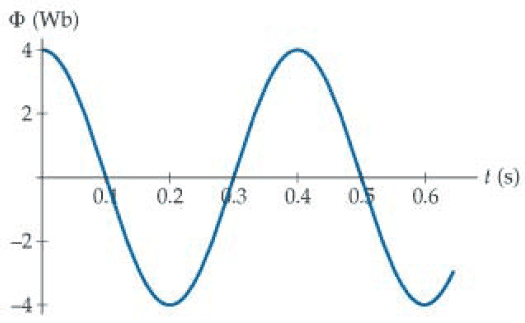# Estimate induced emf in the coil

[Solved] Estimate induced emf in the coil

## Homework Statement

Consider a single-loop coil whose magnetic flux is given by this image:Estimate the induced emf in the coil at times near t = 0.3s, t = 0.4s and t = 0.5s

This is for algebra-based physics.

## Homework Equations

emf = -N*(change in flux)/change in time

## The Attempt at a Solution

At t = 0.3s
emf = -1(4- -4)/(0.4 - 0.2) = -8/0.2 = -40V

At t = 0.4s
Rate of change = 0

At t = 0.5s
emf = -1(-4 - 4)/(0.6 - 0.4) = 8/0.2 = 40V

My first and third answers are wrong. What've I missed? The text book has -0.06kV, 0 and 0.06kV but those answers are also incorrect.

Edit: Solved it. This is the graph of
4 Cos[(2 Pi/(0.4))*x]
Differentiating this gives y = -20pi*Sin[5pi*x]
Evaluating it at t = 0.3 s gives 63V. I do not know why differentiation is used for an algebra-based physics problem when my TA explicitly told me to not use calculus, but there it is.

Last edited:

make a better estimate because the gradient at 0.3s is rather different from the gradient of the line joining the max at 0.4s and the min at 0.2s.

How would I do that?

Using t = 0.25s and t = 0.35s as my points for t = 0.3s,
-(3 - -3)/(0.35 - 0.25) = -6/0.1 = -60V, which is also wrong.

Edit: The correct answer is -63V, 0V, 63V. I have no idea how to calculate this. The textbook has -60V, 0V, 60V, which is apparently incorrect.

Edit: Solved it. This is the graph of
4 Cos[(2 Pi/(0.4))*x]
Differentiating this gives y = -20pi*Sin[5pi*x]
Evaluating it at t = 0.3 s gives 63V. I do not know why differentiation is used for an algebra-based physics problem when my TA explicitly told me to not use calculus, but there it is.

Last edited:
So your second estimate, 60V, was correct!
I do not think you can use differentiation if an estimate was asked for.

Yes, however the homework system won't accept it for some reason. I tried 60V, as the textbook said that, then I tried a wider window and got 40V, then I gave up and fitted it to a cosine curve.

You can get a better estimate than 60V if a tangent is drawn on the curve at the required point and its gradient is calculated. The longer this tangent is drawn the better is the estimate. It is still an estimate since the axes are not subdivided further.

Thanks, I will keep that in mind for next time.